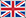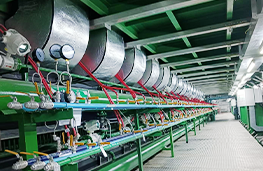Welcome To AsmikEnglishHome > News > A Helpful Guide to Eight Flow Meters

# A Helpful Guide to Eight Flow Meters

2023-02-15

## An Ultimate Guide for Flow Meter Selection

Asmik Meter is a flow meter manufacturer, here has the most complete flow meter selection, and a summary of flow meter knowledge. There are many kinds of flow meters, which are the most widely used flow measurement tools, according to their different characteristics and application fields. Here, Asmik Instruments will briefly introduce the eight commonly used types of flow meters for you. The eight categories to be introduced here are differential pressure flow meter, volumetric flow meter, turbine flow meter, electromagnetic flow meter, ultrasonic flow meter, vortex flow meter, thermal mass flow meter, and Coriolis flow meter.

A flow meter is an instrument used to measure the flow of fluid in a pipeline (the volume of fluid passing per unit of time). There are rotameters, throttling flow meters, slit flow meters, volumetric flow meters, electromagnetic flow meters, ultrasonic flow meters, and weirs. There are many types of flow measurement methods and instruments, and there are many classification methods. So far, there are as many as 60 types of flow meters available for industrial use. The reason why there are so many varieties is that a flow meter that is applicable to any fluid, any range, any flow state, and any use conditions has not been found so far. There are more than 60 kinds of flow meters, and each product has its specific applicability and limitations. According to the measurement object, there are two types: closed pipe and open channel; according to the measurement purpose, it can be divided into total measurement and flow measurement, and the instruments are called total meter and flow meter respectively.

The total meter measures the flow flowing through the pipeline within a period of time, which is represented by the quotient of the total flow in a short period of time divided by the time. In fact, the flow meter is usually equipped with a cumulative flow device, which is used as a total meter. The total meter is also equipped with a flow signaling device. Therefore, it has no practical significance to divide the flow meter and the total meter in a strict sense.According to the measurement principle, there are mechanical principles, thermal principles, acoustic principles, electrical principles, optical principles, atomic physics principles, etc. According to the most popular and extensive classification method at present, it is divided into positive displacement flowmeter, differential pressure flow meter, float flow meter, turbine flow meter, electromagnetic flow meter, vortex flowmeter in the fluid oscillation flow meter, quality Flowmeters, insertion flow meters, probe flow meters are used to explain the principles, characteristics, application profiles and developments at home and abroad of various flow meters.

1. Differential pressure flow meter: This is the most common flow technology, including orifice plates, venturi tubes, and sonic nozzles. DP flow meters can be used to measure the flow rate of most liquids, gases, and steam. DP flow meters have no moving parts and are versatile and easy to use. But after clogging, it will produce pressure loss and affect the accuracy. The accuracy of flow measurement depends on the accuracy of the pressure gauge.
2. Volume flow meter: The flow meter is used to measure the volume flow rate of liquid or gas, it introduces the fluid into the measurement space, and counts the number of rotations. Impellers, gears, pistons, and orifice plates are used to divide fluids. PD flow meters are highly accurate and are one of several methods of measuring viscous liquids. But it also introduces unrecoverable pressure errors and requires moving parts.
3. Turbine flow meter: When the fluid flows through the turbine flowmeter, the fluid makes the rotor rotate. The rotational speed of the rotor is related to the speed of the fluid. The flow rate or total amount is derived from the average flow velocity of the fluid felt by the rotor. Turbine flow meters accurately measure clean liquids and gases. Like PD meters, turbine meters also have unrecoverable pressure errors and also require moving parts.
4. Electromagnetic flow meter: measurement principle, Faraday’s law of electromagnetic induction proves that a conductor moving in a magnetic field will induce an electric potential. Using the principle of electromagnetic measurement, the fluid is a conductor in motion. The induced potential is proportional to the flow velocity and is detected by two measuring electrodes, then the transmitter amplifies it and calculates the flow rate according to the cross-sectional area of the pipe. When a conductive fluid flows through an electromagnetic field, the velocity of the fluid can be obtained by measuring the voltage. Electromagnetic flow meters have no moving parts and are not affected by fluids. Measures conductive liquids with high accuracy when the pipe is full. Electromagnetic flowmeters can be used to measure the flow rate of slurry fluids.
5. Ultrasonic flow meter: The propagation time method and the Doppler effect method are commonly used methods in ultrasonic flowmeters to measure the average velocity of the fluid. Like other speed gauges, it is a meter that measures volumetric flow. It is an unobstructed flow meter and does not need to be inserted if the ultrasonic transmitter is installed outside the pipe. It works with almost all liquids, including slurries, with high precision. But dirt in the pipes can affect accuracy.
6. Vortex flow meter: The vortex flow meter is to place a non-streamlined vortex generator in the fluid, and the speed of the vortex is proportional to the speed of the fluid, so as to calculate the volume flow. Vortex flow meters are suitable for measuring liquids, gases, or steam. It has no moving parts and no dirt issues. Vortex flow meters are noisy and require fluids to have a high flow rate to create vortices.
7. Thermal mass flowmeter: Measure the fluid velocity by measuring the temperature increase of the fluid or the decrease of the thermal sensor. Thermal mass flow meters have no moving parts or holes and accurately measure the flow of gases. Thermal mass flow meters are one of the few technologies that can measure mass flow, and they are also one of the few technologies used to measure large-bore gas flow.
8. Coriolis flowmeter: This flow meter uses a vibrating fluid tube to produce a deflection corresponding to the mass flow rate for measurement. Coriolis flow meters can be used to measure the mass flow of liquids, slurries, gases, or steam. High precision. However, regular maintenance should be carried out on the pipe wall to prevent corrosion.